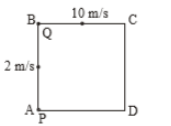You’ve reached the end of your free Videos limit.
#48-Relative motion-3
(Physics) > Motion in A Straight Line
Unable to watch the video, please try another server
Related Practice Questions :

Two men P & Q are standing at corners A & B of square ABCD of side 8 m. They start moving along the track with constant speed 2 m/s and 10 m/s respectively. The time when they will meet for the first time, is equal to:(1) 2 sec

(2) 3 sec

(3) 1 sec

(4) 6 sec

High Yielding Test Series + Question Bank - NEET 2020

Difficulty Level:

Two cars A and B are travelling in the same direction with velocities v1 and v2 $\left({v}_{1}>{v}_{2}\right)$. When the car A is at a distance d behind car B, the driver of the car A applied the brake producing a uniform retardation a. There will be no collision when

1. $d<\frac{{\left({v}_{1}-{v}_{2}\right)}^{2}}{2a}$

2. $d<\frac{{v}_{1}^{2}-{v}_{2}^{2}}{2a}$

3. $d>\frac{{\left({v}_{1}-{v}_{2}\right)}^{2}}{2a}$

4. $d>\frac{{v}_{1}^{2}-{v}_{2}^{2}}{2a}$

High Yielding Test Series + Question Bank - NEET 2020

Difficulty Level:

A student is standing at a distance of 50 metres from the bus. As soon as the bus begins its motion with an acceleration of 1ms–2, the student starts running towards the bus with a uniform velocity u. Assuming the motion to be along a straight road, the minimum value of u, so that the student is able to catch the bus is

1. 5 ms–1

2. 8 ms–1

3. 10 ms–1

4. 12 ms–1

High Yielding Test Series + Question Bank - NEET 2020

Difficulty Level:

A man is 45 m behind the bus when the bus starts accelerating from rest with acceleration of 2.5 m/s2. With what minimum velocity should the man start running to catch the bus?

1. 12 m/s

2. 14 m/s

3. 15 m/s

4. 16 m/s

High Yielding Test Series + Question Bank - NEET 2020

Difficulty Level:

Two trains, each 50 m long are travelling in opposite direction with velocity 10 m/s and 15 m/s. The time of crossing is

(1) 10 s

(2) 4 s

(3) $2\sqrt{3}s$

(4) $4\sqrt{3}s$